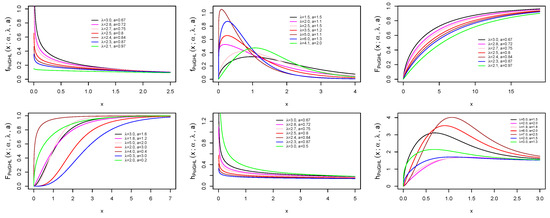Previous Article in Journal
Local-Entropy Based Approach for X-Ray Image Segmentation and Fracture Detection
Open AccessArticle

A New Extension of the Generalized Half Logistic Distribution with Applications to Real Data

College of Mathematics and Information Sciences, Hebei Normal University, Shijiazhuang 050024, China
*
Author to whom correspondence should be addressed.
Entropy 2019, 21(4), 339; https://doi.org/10.3390/e21040339
Received: 13 February 2019 / Revised: 18 March 2019 / Accepted: 25 March 2019 / Published: 28 March 2019
(This article belongs to the Section Information Theory, Probability and Statistics)
In this paper, we introduced a new three-parameter probability model called Poisson generalized half logistic (PoiGHL). The new model possesses an increasing, decreasing, unimodal and bathtub failure rates depending on the parameters. The relationship of PoiGHL with the exponentiated Weibull Poisson (EWP), Poisson exponentiated Erlang-truncated exponential (PEETE), and Poisson generalized Gompertz (PGG) model is discussed. We also characterized the PoiGHL sub model, i.e the half logistic Poisson (HLP), based on certain functions of a random variable by truncated moments. Several mathematical and statistical properties of the PoiGHL are investigated such as moments, mean deviations, Bonferroni and Lorenz curves, order statistics, Shannon and Renyi entropy, Kullback-Leibler divergence, moments of residual life, and probability weighted moments. Estimation of the model parameters was achieved by maximum likelihood technique and assessed by simulation studies. The stress-strength analysis was discussed in detail based on maximum likelihood estimation (MLE), we derived the asymptotic confidence interval of $R = P ( X 1 < X 2 )$ based on the MLEs, and examine by simulation studies. In three applications to real data set PoiGHL provided better fit and outperform some other popular distributions. In the stress-strength parameter estimation PoiGHL model illustrated as a reliable choice in reliability analysis as shown using two real data set. View Full-Text
Show FiguresFigure 1

MDPI and ACS Style

Muhammad, M.; Liu, L. A New Extension of the Generalized Half Logistic Distribution with Applications to Real Data. Entropy 2019, 21, 339.

Show more citation formats Show less citations formats
Note that from the first issue of 2016, MDPI journals use article numbers instead of page numbers. See further details here.

1